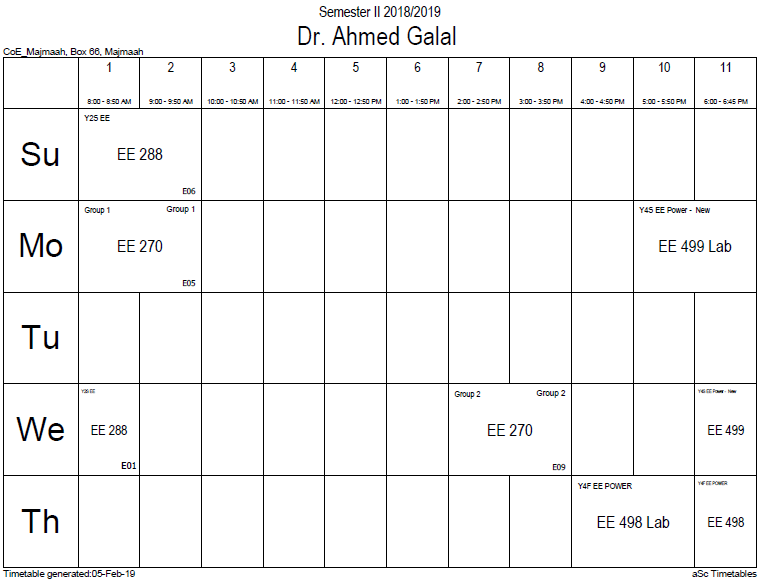## Maxwell's equation

Using the above definition of the potentials and applying it to the other two Maxwell's equations (the ones that are not automatically satisfied) results in a complicated differential equation that can be simplified using the Lorenz gauge where A is chosen to satisfy:$ablacdot extbf{A} + frac{1}{c^2} frac{partial phi}{partial t} = 0.$

Using the Lorenz gauge, Maxwell's equations can be written compactly in terms of the magnetic vector potential A and the electric scalar potential ϕ:$abla^2phi - frac{1}{c^2}frac{partial^2 phi}{partial t^2} = - ho/ epsilon_0$$abla^2 extbf{A} - frac{1}{c^2}frac{partial^2 extbf{A}}{partial t^2} = - mu_0 extbf{J}$

In other gauges, the equations are different. A different notation to write these same equations (using four-vectors) is shown below.

### Office HoursNo office hours### My Timetable### Contactsemail: [email protected]

Phone: 2570

### Welcome

Welcome To Faculty of Engineering### IEEEhttp://www.ieee.org/

/

### Bookmarkshttp://www.utk.edu/research/

http://science.doe.gov/grants/index.asp

http://www1.eere.energy.gov/vehiclesandfuels/

http://www.eere.energy.gov/

### Upcoming Conferences### Engineering quotes### Travel Web Siteshttp://www.hotels.com/

http://www.orbitz.com/

http://www.hotwire.com/us/index.jsp

http://www.kayak.com/

### Blackboardستقام اختبارات الميدتيرم يوم الثلاثاء 26-6-1440

حسب الجدول المعلن بلوحات الاعلان

### Summer trainingThe registration for summer training will start from 5th week of second semesterClass registration week 1

### برنامج التجسير### إحصائية الموقع

عدد الصفحات: 2879

البحوث والمحاضرات: 1280

الزيارات: 101048